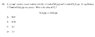# Balanced chemical equation

• Hevonen

## Homework Statement

A 1.0 dm3 reaction vessel contains initially 1.0 mol of N2O4(g) and 1.0 mol of NO2(g).
At equilibrium, 0.75mol of N2O4(g) are present.
What is the value of Kc?
A. 0.33
B. 0.50
C. 2.0
D. 3.0

## The Attempt at a Solution

Right answer = D, but I do not why. My attempt for Kc is (3/4)^2 / (9/8) = 0.5, so wrong answer. Please, help me with this stricky one.

#### Attachments

•phy.JPG
13.1 KB · Views: 395
Last edited:
Show the equilibrium equation in terms of the agents and also the actual balanced chemical equation.

Show the equilibrium equation in terms of the agents and also the actual balanced chemical equation.

Yes indeed. That is the right method to solve this.

Thank you!